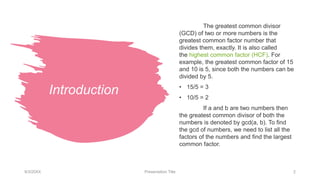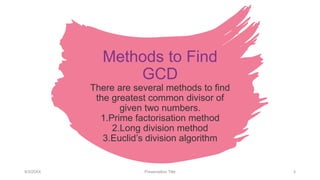Successfully reported this slideshow.

# Greatest Common Divisor (GCD).pptx×

# Greatest Common Divisor (GCD).pptx

The greatest common divisor (GCD) of two or more numbers is the greatest common factor number that divides them, exactly. It is also called the highest common factor (HCF).
How to find the greatest common divisor
Before we move ahead on finding the greatest common factor of numbers, let us understand what a factor and common factors are?

What are Factors?
Factors of a number divides the original number evenly. For example, if 8 is the factor of 64, then 8 can divide 64 into 8 equal parts.

What are Common Factors?
If factor of a number is a factor of another number, then it is said to be a common factor for the two numbers. For example, 2 is a factor of 4 and 8, hence 2 is a common factor.

GCD
GCD is the greatest common factor of two or more numbers. A factor that is the highest among the numbers.

Methods to Find GCD
There are several methods to find the greatest common divisor of given two numbers.

Prime factorisation method
Long division method
Euclid’s division algorithm

The greatest common divisor (GCD) of two or more numbers is the greatest common factor number that divides them, exactly. It is also called the highest common factor (HCF).
How to find the greatest common divisor
Before we move ahead on finding the greatest common factor of numbers, let us understand what a factor and common factors are?

What are Factors?
Factors of a number divides the original number evenly. For example, if 8 is the factor of 64, then 8 can divide 64 into 8 equal parts.

What are Common Factors?
If factor of a number is a factor of another number, then it is said to be a common factor for the two numbers. For example, 2 is a factor of 4 and 8, hence 2 is a common factor.

GCD
GCD is the greatest common factor of two or more numbers. A factor that is the highest among the numbers.

Methods to Find GCD
There are several methods to find the greatest common divisor of given two numbers.

Prime factorisation method
Long division method
Euclid’s division algorithm

### Greatest Common Divisor (GCD).pptx

1. 1. Greatest Common Divisor (GCD) VAISHIKA G
2. 2. Introduction 9/3/20XX Presentation Title 2 The greatest common divisor (GCD) of two or more numbers is the greatest common factor number that divides them, exactly. It is also called the highest common factor (HCF). For example, the greatest common factor of 15 and 10 is 5, since both the numbers can be divided by 5. • 15/5 = 3 • 10/5 = 2 If a and b are two numbers then the greatest common divisor of both the numbers is denoted by gcd(a, b). To find the gcd of numbers, we need to list all the factors of the numbers and find the largest common factor.
3. 3. 9/3/20XX Presentation Title 3 Methods to Find GCD There are several methods to find the greatest common divisor of given two numbers. 1.Prime factorisation method 2.Long division method 3.Euclid’s division algorithm
4. 4. Prime factorisation method 9/3/20XX Presentation Title 4 • Every composite number, i.e. a number with more than one factor can be written as a product of prime numbers. • In the prime factorisation method, each given number is written as the product of prime numbers and then find the product of the smallest power of each common prime factor. • This method is applicable only for positive numbers, i,e. Natural numbers. Example: Find the Greatest common factor of 24, 30 and 36. Solution: Prime factors of 24 is 23 × 3 Prime factors of 30 = 2 × 3 × 5 Prime factors of 36 = 2² x 3² From the factorisation, we can see, only 2 x 3 are common prime factors. Therefore, GCD (24, 30, 36) = 2 x 3 = 6
5. 5. Long division method In this method, the largest number among the given set of numbers should be divided by the second largest number, and again the second-largest number should be divided by the remainder of the previous operation, this process will continue till the remainder is zero. The divisor, when the remainder is zero, is called the greatest common divisor of the given numbers. 9/3/20XX Presentation Title 5
6. 6. Formula If a and b are any number, then the greatest common divisor of a and b can be given by: 𝐺𝐶𝐷 𝑎, 𝑏 = [|𝑎. 𝑏|] [𝑙𝑐𝑚(𝑎, 𝑏)] Presentation Title 6
7. 7. Application • The concept of the greatest common divisor or the highest common factor is used in many real-life incidents as below. • A shopkeeper has 420 balls and 130 bats to pack in a day. She wants to pack them in such a way that each set has the same number in a box, and they take up the least area of the box. What is the number that can be placed in each set for this packing purpose? • In the above problem, the greatest common divisor of 420 and 130 will be the required number. • Other application like arranging students in rows and columns in equal number, diving a group of people into smaller sections,etc., 9/3/20XX Presentation Title 7
8. 8. Example 1: Find the greatest common divisor (or HCF) of 128 and 96. By the method of prime factorisation, • 128 = 2 x 2 x 2 x 2 x 2 x 2 x 2 • 96 = 2 x 2 x 2 x 2 x 2 x 3 • HCF (128, 96) = 2 x 2 x 2 x 2 x2 = 32 9/3/20XX Presentation Title 8 By the method of division, Hence, 32 is the HCF of 128 and 96.
9. 9. Example 2: Two rods are 22 m and 26 m long. The rods are to be cut into pieces of equal length. Find the maximum length of each piece. • HCF or GCD is the required length of each piece. • 22 = 2 x 11 • 26 = 2 x 13 • HCF or the greatest common divisor = 2 • Hence, the required maximum length of each piece is 2 m. 9/3/20XX Presentation Title 9
10. 10. Example 3: Find the greatest common factor of 24, 148 and 36. • Prime factorisation of given numbers is • 24 = 2 × 2 × 2 × 3 • 148 = 2 × 2 × 37 • 36 = 2 × 2 × 3 × 3 • Greatest common factor = 2 × 2 = 4 9/3/20XX Presentation Title 10
11. 11. Thank you VAISHIKA G

### Editor's Notes

• (These don’t have designer IDs since they were based off the default master slides already in the deck.